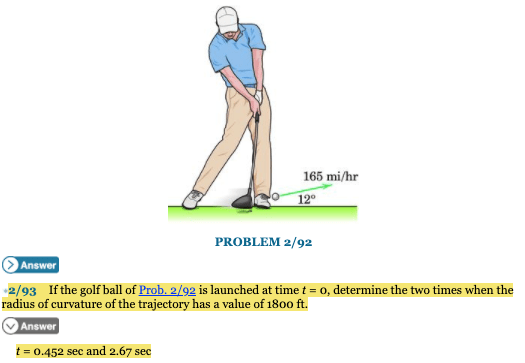# If the golf ball of Prob. 2/92 is launched at time t = 0, determine the two times when the radius of curvature of the trajectory has a value of 1800 ft

Question-AnswerCategory: PhysicsIf the golf ball of Prob. 2/92 is launched at time t = 0, determine the two times when the radius of curvature of the trajectory has a value of 1800 ft

If the golf ball of Prob. 2/92 is launched at time t = 0, determine the two times when the radius of curvature of the trajectory has a value of 1800 ft.